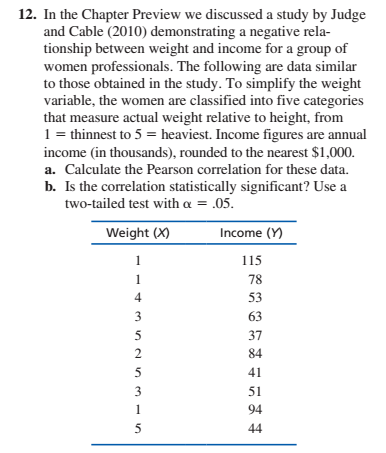# 12. In the Chapter Preview we discussed a study by Judgeand Cable (2010) demonstrating a negative rela-tionship between weight and income for a group ofwomen professionals. The following are data similarto those obtained in the study. To simplify the weightvariable, the women are classified into five categoriesthat measure actual weight relative to height, from1 = thinnest to 5 = heaviest. Income figures are annualincome (in thousands), rounded to the nearest \$1,000.a. Calculate the Pearson correlation for these data.b. Is the correlation statistically significant? Use atwo-tailed test with a = .05.Weight (X)Income (Y)115781453363378454135194144

Question
4 viewshelp_outlineImage Transcriptionclose12. In the Chapter Preview we discussed a study by Judge and Cable (2010) demonstrating a negative rela- tionship between weight and income for a group of women professionals. The following are data similar to those obtained in the study. To simplify the weight variable, the women are classified into five categories that measure actual weight relative to height, from 1 = thinnest to 5 = heaviest. Income figures are annual income (in thousands), rounded to the nearest \$1,000. a. Calculate the Pearson correlation for these data. b. Is the correlation statistically significant? Use a two-tailed test with a = .05. Weight (X) Income (Y) 115 78 1 4 53 3 63 37 84 5 41 3 51 94 1 44 fullscreen
check_circle

Step 1

Correlation coefficient - r:

The Karl Pearson’s product-moment correlation coefficient or simply, the Pearson’s correlation coefficient is a measure of the strength of a linear association between two variables and is denoted by r or rxy.

The coefficient of correlation rxy between two variables x and y for the bivariate data set (xi, yi) for i=1,2,3…N is given below:

Step 2

The Pearson’s product-moment correlation does not take into consideration whether a variable has been classified as a dependent or independent variable. It treats all variables equally. A change of scale and origin does not affect the value of r. Indeed, the calculations of Pearson’s correlation coefficient were designed in such that the units of measurement do not affect the calculation. This allows the correlation coefficient to be comparable and not influenced by the units of the variables used.

From the above said points, it is clear that, rxy = ryx. In the formula for r, if we exchange the symbols x and y, then the result is same. This is because the formula is not dependent on the symbols.

a)

Let r denote the linear correlation between Weight (X) and Income (Y).

Sample correlation coefficient:

The value of the sample correlation coefficient (r) can be obtained using Excel formula.

Enter the values of weight in columns A2:A11 in Excel.

Enter the values of income in columns B2:B11 in Excel.

Enter the fun...

### Want to see the full answer?

See Solution

#### Want to see this answer and more?

Solutions are written by subject experts who are available 24/7. Questions are typically answered within 1 hour.*

See Solution
*Response times may vary by subject and question.
Tagged in

### Other